Engineer Talk

# Heat Transfer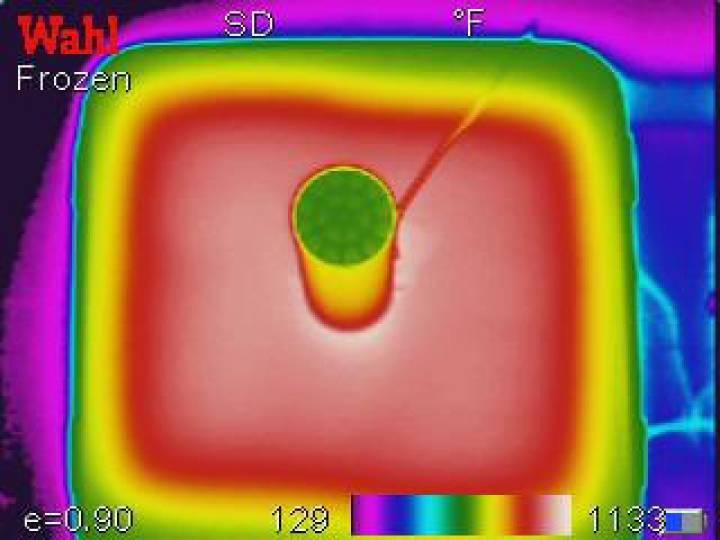Thermodynamics, specifically heat transfer, is used throughout our daily lives, but not always thought of.  A common practice of cooking breakfast would be one simple example.  You place one type of media, your frying pan, onto a hot surface and apply the “heat”, which is your source of energy, to cook the food.  The heat transfer that is occurring between the higher temperature stove-top and the cooler frying pan is a great practical application.  It is also a simple example of the second law of thermodynamics, “Heat cannot, of itself, pass from a lower temperature to a higher temperature.” Thus, for heat transfer to occur, we can state that a temperature difference must exist between two mediums.

As we further study heat transfer and any thermal system, we will need to consider the three types of heat transfer, which are conduction, convection, and radiation.  In many cases you can have two or even all three sources of heat transfer happening simultaneously.   As each form of heat transfer is briefly discussed I will list an example in the following paragraphs.

In order to fully understand how conduction, convection, and radiation are affected, you must consider the rate at which a certain medium will affect your system design.  The rate at which energy or heat is absorbed or dissipated is dictated by the thermal conductivity of a material or combination of materials, temperature difference, area of the surfaces, and mass of the combined components.  By varying the previously mentioned attributes, one can increase or decrease the speed and efficiency of a thermal system.

Now let’s look at a short study of each type of heat transfer.  The first form is conduction.  Conduction is a thermal process that occurs between two surfaces in contact with each other, where a temperature gradient exists, or even in one material that has a temperature gradient between two planes.  If we use a simple experiment of a uniform bar of cross-section A, perfectly insulated on all sides except at the ends; where heat can only flow in the x direction (see Fig. 1).  If the bar is maintained at t1 on one end and t2 at the other end, Q (BTU/Hr (BTU = British Thermal Units, HR = Hours) will be transferred steadily from the entry, at location 1 to the exit at location 2.  The rate of heat flow (heat flux) is directly proportional to the cross-sectional area and temperature difference from point to point of the bar.  You may want to compare the cross-sectional relationship of the bar to how water flows through a pipe.  The larger diameter of the pipe, the more flow of water (energy) it can transfer.  If we now determine how the length of the bar will affect the heat transfer rate, we double the length (2L).  It is found that the heat transfer rate is cut in half, which demonstrates that the heat transfer is inversely proportional to the length of the bar.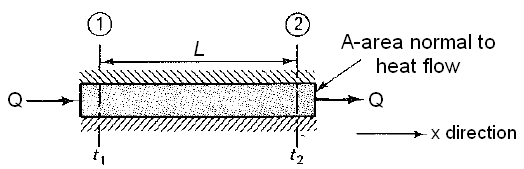Fig. 1

Equation 1 shows mathematically the relationship of all the factors, where the proportionality constant, k, is a property of the material called thermal conductivity.  The negative sign has been included in equation 1 to indicate a positive heat flow. The conductivity, k, is usually a function of temperature, but for moderate temperatures and temperature differences, it can be considered a constant.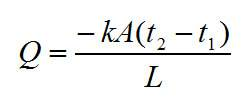Equation 1: Reference bibliography

Example:
A plane wall constructed of solid iron with thermal conductivity 70 W/m°C, thickness 50 mm and with surface area 1 m by 1 m, temperature 150°C on one side and 80°C on the other.

Conductive heat transfer can be calculated as:

q = 70 (W/m°C) 1 (m) 1 (m) (150 (°C) - 80 (°C)) / 0.05 (m)

= 98,000 (W)

= 98 (kW)

The second form of heat transfer is convection.  Convection is the transfer of heat through motion of a liquid or gas relative to the body of material.   There are two types of convection, forced convection and natural convection.  If the motion of fluid is caused by the different densities initiated by the different temperatures in various locations of a fluid, it is known as natural convection.  If the motion of the fluid is caused by an external force, such as a fan or blower in air heating, than it is considered forced convection.  With natural convection, the minor temperature differences in a fluid can cause the heat transfer.  For example, a room in your house could have small temperature differences from an outside wall to an interior wall.  Those hot and cold particles coming into contact with the wall will collide and cause a transfer of energy.  The equation for Newton’s law of cooling helps explain how basic convection is mathematically represented (see Equation 2).   It is much more in depth to explain forced convection, so that could be covered in future articles.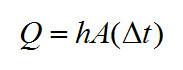Equation 2: Reference Bibliography

Q = heat-transfer rate (BTU/hr)

A = heat-transfer area (FT2)

∆T = temperature difference between the surface and the bulk of the fluid away from the surface (°F)

h = coefficient of heat transfer  (BTU/hr - ft² - °F)

Example:
A fluid flows over a plane surface 1 m by 1 m with a bulk temperature of 50°C. The temperature of the surface is 20°C. The convective heat transfer coefficient is 2,000 W/m2°C.

Q = 2,000 (W/m2°C) (1 (m) 1 (m)) (50 (°C) - 20 (°C))

= 60,000 (W)

= 60 (Kw)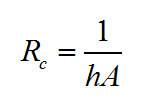Equation 3 - Thermal Resistance for Convection

Rc = Convective heat transfer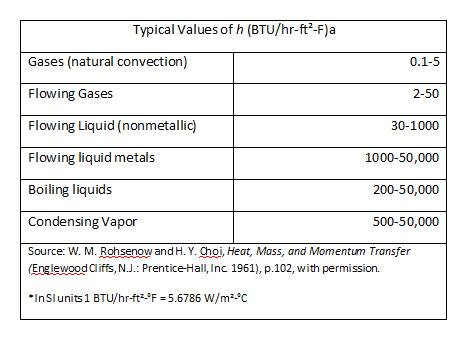Table 1

The third and final form of heat transfer is radiation.  Radiant heat transfer differs from the other forms.  Radiation does not require any mediums to transfer heat.  Radiant heat transfer is similar to the “electromagnetic phenomenon” similar to light, x-rays, and radio waves.1 In this case a transfer of heat occurs when the absorption of energy is greater that what is radiating from the same body.  A body that absorbs all radiation and does not radiate any heat energy itself is considered a “blackbody.”  The small amount of heat that is reflected is considered the body’s reflectivity, the amount of heat absorbed is known as absorptivity, and the effectiveness of as a thermal radiator is known as emmissivity.  The radiant heat transfer rate is shown in equation 4.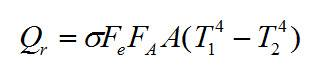Equation 4

σ = Stefan-Boltzmann constant = 0.173 x 10 - 8 BTU/hr - ft2 - °R4 (in SI – 5.669 x 10-8 Watts/m2 - °K4)

Fe = emissivity factor

FA = Geometric factor to allow for the average solid angle through which one surface “sees” the other

A = area, Ft2 (in SI, M2)

T1, T2 = absolute temperatures, °R (Rankin) (In SI, °K (Kelvin))

Example: Radiation from the surface of the Sun
If the surface temperature of the sun is 5800 K and if we assume that the sun can be regarded as a black body the radiation energy per unit time can be expressed by modifying (1) like

q / A = σ T4

= 5.6703 10-8 (W/m2K4) (5800 (K))4

= 6.42 107 (W/m2)

All three of the previously mentioned heat transfer factors must be considered when sizing a heater for any application.  If a band, cartridge, or a strip heater, is selected, all of these elements work on the same design principles.  The system can be insulated to improve efficiency during operation and controlled to more accurately provide heat.  The final power requirements and efficiencies will depend on a well designed system that eliminates heat loss and offers close control.  A good rule of thumb, after the initial requirements are determined, that a designer uses a 25% service factor, or a 1.25 multiplier for the wattage output of the system.

Bibliography
Equation 1, 2, 3 and Table 1 from “Thermodynamics and Heat Power”, Sixth Edition by Irving Granet and Maurice Bluestein, Copywrite 2000, Published by Prentice-Hall, Inc., Upper Saddle River, New Jersey 07458

Footnotes
1 See page 581, from “Thermodynamics and Heat Power”, Sixth Edition by Irving Granet and Maurice Bluestein, Copy-write 2000, Published by Prentice-Hall, Inc., Upper Saddle River, New Jersey 07458

Tutco-Farnam Custom Products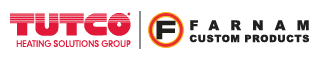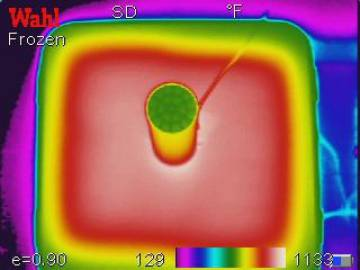Calculators
Calculators +